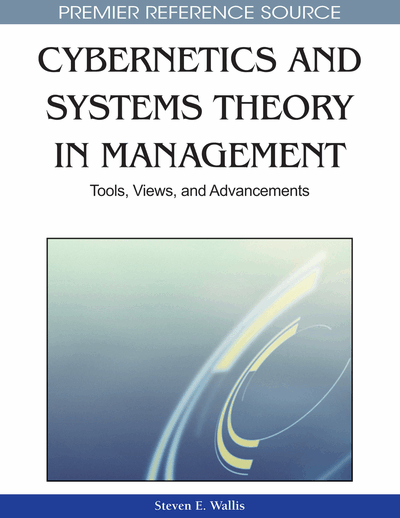# Selection of the Best Subset of Variables in Regression and Time Series Models

Nicholas A. Nechval (University of Latvia, Latvia), Konstantin N. Nechval (Transport and Telecommunication Institute, Latvia), Maris Purgailis (University of Latvia, Latvia) and Uldis Rozevskis (University of Latvia, Latvia)
DOI: 10.4018/978-1-61520-668-1.ch016
Available
\$37.50
No Current Special Offers

## Abstract

The problem of variable selection is one of the most pervasive model selection problems in statistical applications. Often referred to as the problem of subset selection, it arises when one wants to model the relationship between a variable of interest and a subset of potential explanatory variables or predictors, but there is uncertainty about which subset to use. Several papers have dealt with various aspects of the problem but it appears that the typical regression user has not benefited appreciably. One reason for the lack of resolution of the problem is the fact that it is has not been well defined. Indeed, it is apparent that there is not a single problem, but rather several problems for which different answers might be appropriate. The intent of this chapter is not to give specific answers but merely to present a new simple multiplicative variable selection criterion based on the parametrically penalized residual sum of squares to address the subset selection problem in multiple linear regression analysis, where the objective is to select a minimal subset of predictor variables without sacrificing any explanatory power. The variables, which optimize this criterion, are chosen to be the best variables. The authors find that the proposed criterion performs consistently well across a wide variety of variable selection problems. Practical utility of this criterion is demonstrated by numerical examples.
Chapter Preview
Top

## Introduction

Variable selection refers to the problem of selecting input variables that are most predictive of a given outcome. Variable selection problems are found in all supervised or unsupervised machine learning tasks, classification, regression, time series prediction, pattern recognition.

In the recent years, variable selection has become the focus of considerable research in several areas of application for which datasets with tens or hundreds of thousands of variables are available. These areas include text processing, particularly in application to Internet documents, and genomics, particularly gene expression array data. The objective of variable selection is three-fold: to improve the prediction performance of the predictors, to provide faster and more cost-effective predictors, and to provide a better understanding of the underlying process that generated the data.

A number of studies in the statistical literature discuss the problem of selecting the best subset of predictor variables in regression. Such studies focus on subset selection methodologies, selection criteria, or a combination of both. The traditional selection methodologies can be enumerative (e.g. all subsets and best subsets procedures), sequential (e.g. forward selection, backward elimination, stepwise regression, and stagewise regression procedures), and screening-based (e.g. ridge regression and principal components analysis). Standard texts like Draper and Smith (1981) and Montgomery and Peck (1992) provide clear descriptions of these methodologies.

Some of the reasons for using only a subset of the available predictor variables (given by Miller, 2002) are:

• • To estimate or predict at a lower cost by reducing the number of variables on which data are to be collected;

• • To predict more accurately by eliminating uninformative variables;

• • To describe multivariate data sets parsimoniously; and

• • To estimate regression coefficients with smaller standard errors (particularly when some of the predictors are highly correlated).

These objectives are of course not completely compatible. Prediction is probably the most common objective, and here the range of values of the predictor variables for which predictions will be required is important. The subset of variables giving the best predictions in some sense, averaged over the region covered by the calibration data, may be very inferior to other subsets for extrapolation beyond this region. For prediction purposes, the regression coefficients are not the primary objective, and poorly estimated coefficients can sometimes yield acceptable predictions. On the other hand, if process control is the objective then it is of vital importance to know accurately how much change can be expected when one of the predictors changes or is changed.

Suppose that y, a variable of interest, and x1, ..., xv, a set of potential explanatory variables or predictors, are vectors of n observations. The problem of variable selection, or subset selection as it is often called, arises when one wants to model the relationship between y and a subset of x1, ..., xv, but there is uncertainty about which subset to use. Such a situation is particularly of interest when v is large and x1, ..., xv is thought to contain many redundant or irrelevant variables.

The variable selection problem is most familiar in the linear regression context, where attention is restricted to normal linear models. Letting w index the subsets of x1, ..., xv and letting pw be the number of the parameters of the model based on the wth subset, the problem is to select and fit a model of the formy = Xwaw + ε, (1) where Xw is an n × pw matrix whose columns correspond to the wth subset, aw is a pw × 1 vector of regression coefficients, and ε~Nn(02I). More generally, the variable selection problem is a special case of the model selection problem where each model under consideration corresponds to a distinct subset of x1, ..., xv. Typically, a single model class is simply applied to all possible subsets.

## Complete Chapter List

Search this Book:
Reset